# Regression Analysis 201 - Stepwise Regression

This is the second entry in our regression analysis and modeling series. In this tutorial, we continue the analysis discussion we started earlier and leverage an advanced technique – stepwise regression in Excel - to help us find an optimal set of explanatory variables for the model.

Again, we will use a sample data set gathered from 20 different salespersons. The regression model attempts to explain and predict weekly sales for each salesperson (dependent variable) using two explanatory variables: intelligence (IQ) and extroversion.

## Data Preparation

Similar to what we did in an earlier tutorial, we organize our sample data by placing the value of each variable in a separate column and each observation in a separate row.

Next, we introduce the “mask.” The “mask” is a Boolean array (0,1), which chooses which variable is included (or excluded) from the analysis.

Initially, at the top of the table, let’s insert the mask cells array, each with a value of 1 (i.e. included). The array is shown highlighted below.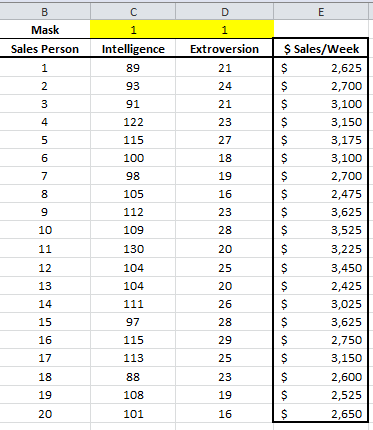In this example, we have 20 observations and two independent (explanatory) variables. The response or dependent variable is the weekly sales.

## Process

Now, we are ready to conduct our regression analysis. First, select an empty cell in your worksheet where you wish the output to be generated, then locate and click on the regression icon in the NumXL tab (or toolbar).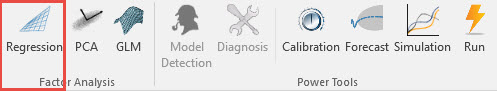The Regression Wizard appears.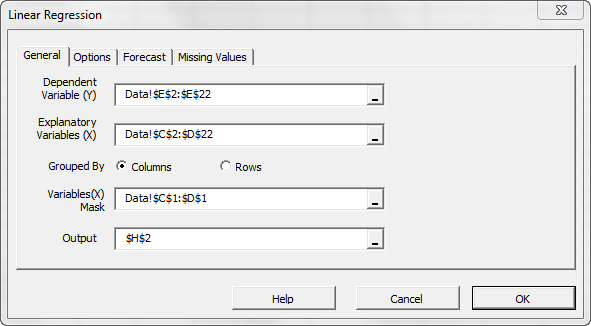Select the cell range for the response/dependent variable values (i.e. weekly sales). Select the cell range for the explanatory (independent) variables values. For “Variables (X) Mask,” select the cells at the top of the data table (Boolean array).

Notes:

1. The cells range includes (optional) the heading (“Label”) cell, which would be used in the output tables where it references those variables.
2. The explanatory variables (i.e. X) are already grouped by columns (each column represents a variable), so we don’t need to change that.
3. By default, the output cells range is set to the currently selected cell in your worksheet.

Please note that once we select the X and Y cells range, the “Options,” “Forecast” and “Missing Values” tabs become available (enabled).

Next, select the “Options” tab.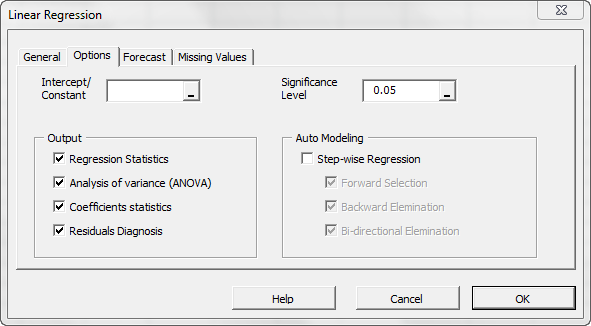Initially, the tab is set to the following values:

• The regression intercept/constant is left blank. This indicates that the regression intercept will be estimated by the regression. To set the regression to a fixed value (e.g. zero (0)), enter it there.
• The significance level (aka. \alpha ) is set to 5%.
• In the “Output” section, the most common regression analyses are selected.
• Leave “Auto Modeling” unchecked. We will discuss this functionality in a later issue.

Now, click on the “Missing Values” tab.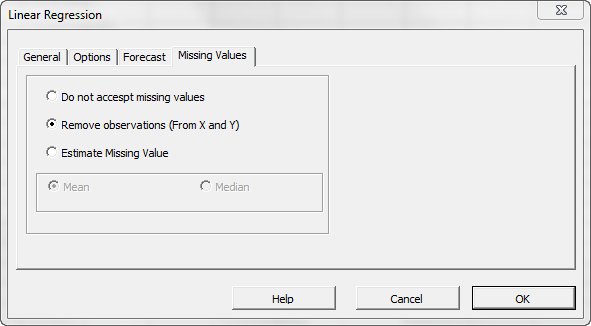In this tab, you can select an approach to handle missing values in the data set (X and Y). By default, any missing value found in X or in Y in any observation would exclude the observation from the analysis.

This treatment is a good approach for our analysis, so let’s leave it unchanged.

Now, click “OK” to generate the output tables: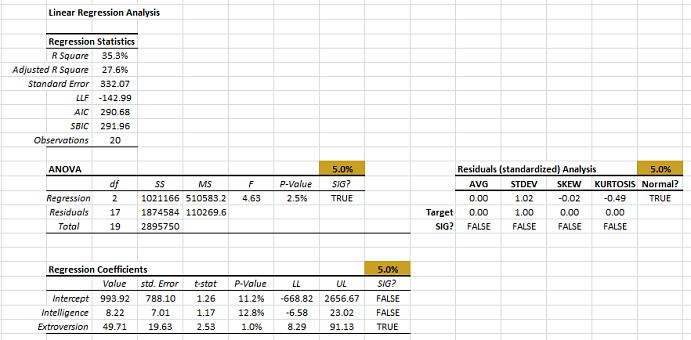## Analysis

Aside from the “Variables (X) Mask” settings, everything is exactly the same as we did in the prior tutorial, so what’s our next step?

The “Mask” variable determines which variable is included in the regression analysis, so let’s take another look at the “Coefficients” table.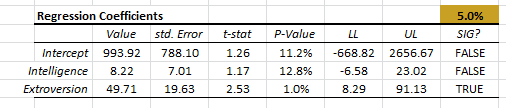First, let’s exclude the “Intelligence” input variable from the analysis. This is done simply by flipping the mask value for this cell to zero.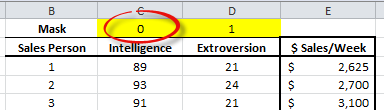Now, if you have the “Calculation” option set to manual, force recalculation. Otherwise, the spreadsheet recalculates automatically.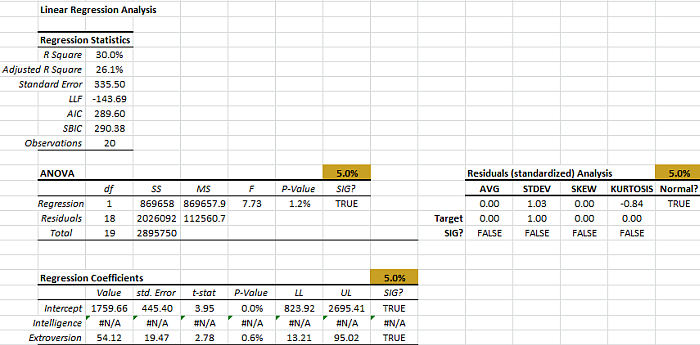Checking the output tables, we find the following:

• R square dropped by 6%.
• Adjusted R square dropped by 1.5%.
• Standard error increased by 3.
• AIC dropped by one (1).
• ANOVA table shows the regression is significant.
• Residual diagnosis checks out for all tests.
• In the regression coefficients table, the intercept and the coefficient of the “Extroversion” variable are both statistically significant.

This model has fewer parameters (i.e. one) and explains the variation in the values of the response variable just as well as when we had two (2) explanatory variables.

Now, let’s plot the estimated values against the actual.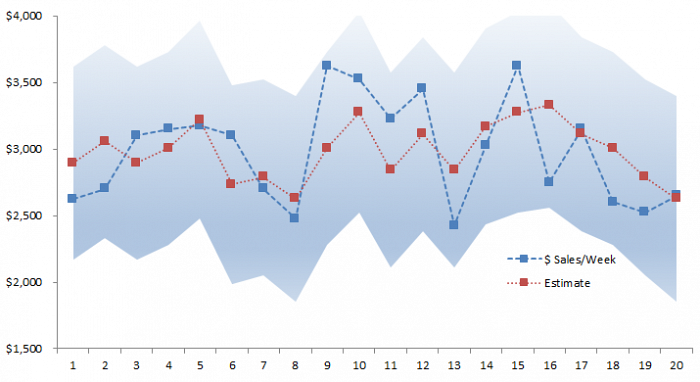The shaded area represents the 95% confidence interval for the estimates of the regression model.

So far, we have demonstrated that dropping a variable from the analysis is as easy as flipping a switch; no more copying data and cluttering your spreadsheet with tons of output tables. This is nice, but you might be wondering: if I had more explanatory variables (say 10), what is the optimal set of variables? Should I try every single subset?

NumXL supports an interesting functionality – stepwise regression in Excel – to help you select this optimal set. Let’s demonstrate how you would use it.

1. In the “Mask” cells range, turn the variables on or off that you wish the stepwise regression in Excel to consider. For this demonstration, we will turn them all on.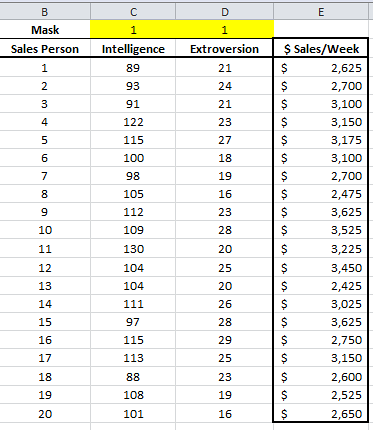2. Locate and click on the regression icon in the NumXL tab.
3. The Regression Wizard pops up.
4. In the “General” tab, select the input cells range and the mask cells range.
5. Under the Options tab, check the “Step-wise Regression” box.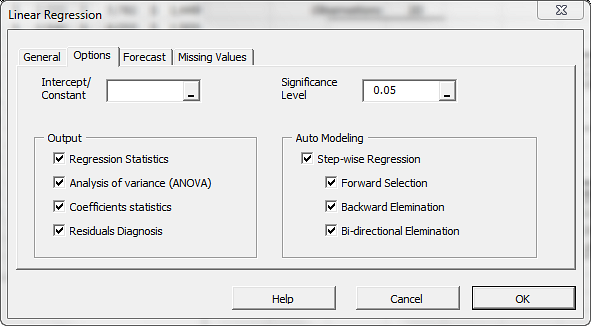6. Leave the 3 different methods checked.
7. Click “OK.”
8. The output tables are generated.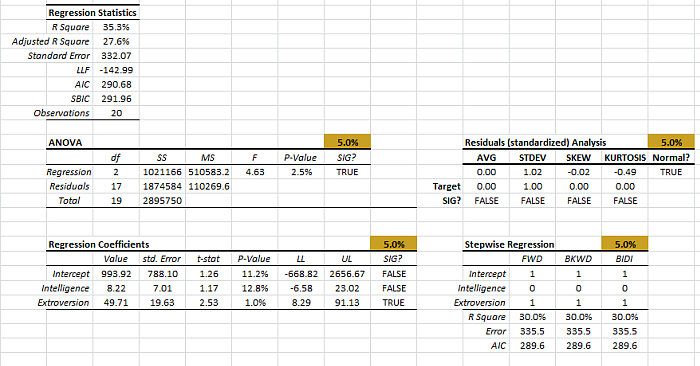The stepwise regression in Excel generates one additional table next to the coefficients table.

Let’s take a closer look at this new table.

The stepwise regression carries on a series of partial F-test to include (or drop) variables from the regression model.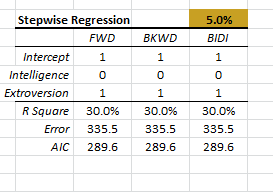• Backward elimination: we start from the full model with all variables in, and consider dropping one repressor at a time.
• Bi-directional elimination is a hybrid of the two methods.

The table displays the “mask” for the optimal model found in each column. One (1) stands for inclusion and zero (0) for exclusion.

At the bottom of the table, we compute the regression statistics for each model for our comparison. In this case, the three models came back with the same set of variables, so no comparison is needed.

Please note that, given the same set of input variables and responses, the “mask” is used to differentiate one model from others simply by listing the inclusion/exclusion list.

## Conclusion

So far, we have created a regression model, examined its significance, verified that it satisfies underlying assumptions, and found the optimal subset of variables of the model.

For many, this is the end of the analysis, and they would probably start using it for forecasting.

Before we can use the model for forecasting, there are two more questions we ought to answer:

1. Do we have any observation that exerts a significant influence (e.g. outliers) on the regression model?
2. Is the regression model stable over the sample data?

This will be covered in the 3rd entry in our regression tutorial series. Please, read on.